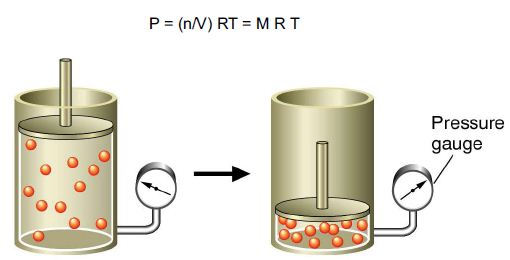# Describe on Equilibrium Constant Kp

If we express a gas-phase equilibria in terms of partial pressures, we obtain the equilibrium constant Kp. For the reaction below:

CO (g) + 3H2 (g) = CH4 (g) + H2O (g)

The equilibrium-constant expression in terms of partial pressures becomes:

KP = (PCH4 PH2O)/(PCO PH2)In general, the numerical value of Kp is not the same as the value of Kc. From the relationship P V = n R T, we show that

Kp = Kc (RT)Δn

Where: An is the total amount of moles of gaseous product LESS the total amount of moles of gaseous reactant. Consider the following reaction:

2SO2 (g) + O2 (g) ↔ 2SO3 (g)

Reaction constant Kc for the reaction is 2.8 x 102 at 1,000 °C. Calculate Kp for the reaction at this temperature.

We know that: Kp = Kc (RT)Δn

From the equation we see that Δn = 2 – (2 +1) = -1. We can simply substitute the given reaction temperature and the value of R (0.08206 dm3.atm/molK) to obtain Kp.

Thus,

Kp = 2.8 x 102 x (0.08206 x 1,000)-1

Kp = 3.4